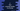# C program to find the missing number in an array## How to find the missing number in an array in C:

In this post, we will learn how to find the missing number in an array. The array will hold all numbers from 1 to n with one number missed. Our program will print the missing number.

For example, if the array is [1, 2, 3, 4, 5, 7], the missing number is 6. We can have the array elements in any order.

We can solve this problem in different ways. I will show you how to do that.

### Method 1: By finding the sum of all numbers:

The sum of all numbers from 1 to n is n * (n + 1)/2. So, if the array size is n, and since one number is missing from the array, we can find the total sum as (n + 1) * (n + 2)/2. If we calculate the sum of all numbers in the array and subtract that from the total sum, it will give the missing number.

Below is the complete program:

``````#include <stdio.h>

int findMissingNumber(int arr[], int size)
{
int totalSum = (size + 1) * (size + 2) / 2;
int i, currentSum = 0;

for (i = 0; i < size; i++)
{
currentSum += arr[i];
}

}

int main()
{
int i, n;
printf("Enter the size of the array : ");
scanf("%d", &n);

int arr[n - 1];

printf("Enter the values : \n");
for (i = 0; i < n; i++)
{
scanf("%d", &arr[i]);
}

int missingNumber = findMissingNumber(arr, n);

printf("Missing number is: %d\n", missingNumber);
return 0;
}``````

Here,

• The program is reading the array elements and keeping it in the array arr.
• findMissingNumber method is used to find the missing number. This is the main method where we are doing the actual part.
• Inside findMissingNumber, we are first calculating the total sum of the array elements. This is the sum of all numbers if we have all the numbers in the array including the missing number.
• currentSum is the sum of all the current numbers in the array.
• So, totalSum - currentSum gives the missing number in the array.

It will give output as like below:

``````Enter the size of the array : 7
Enter the values :
1 2 3 4 5 7 8
Missing number is: 6``````

### Method 2: Using XOR:

We can also solve this by using XOR. For example, if the array has numbers from 1 to n and one value is missing here, then,

if XOR of all numbers from 1 to n = a, XOR of all numbers from 1 to n without the missing number = b, then a XOR b will be the missing number.

So,

• The program will run one loop from 1 to n and find the XOR of all numbers, and keep that value in a variable say firstXOR.
• It will run one second loop and find the XOR of all numbers in the given array and store that value in a different variable, secondXOR.
• XOR or firstXOR and secondXOR will give the missing number.

Below is the complete program:

``````#include <stdio.h>

int findMissingNumber(int arr[], int size)
{
int i;
int firstXOR = 1;
int secondXOR = arr;

for(i = 2; i<= size + 1; i++)
firstXOR = firstXOR ^ i;

for(i = 1; i< size; i++)
secondXOR = secondXOR ^ arr[i];

return firstXOR ^ secondXOR;
}

int main()
{
int i, n;
printf("Enter the size of the array : ");
scanf("%d", &n);

int arr[n - 1];

printf("Enter the values : \n");
for (i = 0; i < n; i++)
{
scanf("%d", &arr[i]);
}

int missingNumber = findMissingNumber(arr, n);

printf("Missing number is: %d\n", missingNumber);
return 0;
}``````

Here,

• we are changing only the findMissingNumber method.
• The first for loop finds the XOR of all numbers from 1 to n. The second loop finds the XOR or all elements in the array.
• It returns the XOR of both results.

If you run this program, it will give similar output.

``````Enter the size of the array : 7
Enter the values :
1 3 4 5 6 7 8
Missing number is: 2``````